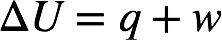# Problem: Calculate the internal energy change for each of the following. A piston is compressed from a volume of 8.30 L to 2.80 L against a constant pressure of 1.90 atm. In the process, there is a heat gain by the system of 350. J.

###### FREE Expert Solution

Recall: The change in internal energy ΔU is given by:where q = heat and w = work.

We're given q = 350 J. This has a positive sign since heat was gained by the system.

80% (402 ratings)###### Problem Details

Calculate the internal energy change for each of the following.

A piston is compressed from a volume of 8.30 L to 2.80 L against a constant pressure of 1.90 atm. In the process, there is a heat gain by the system of 350. J.